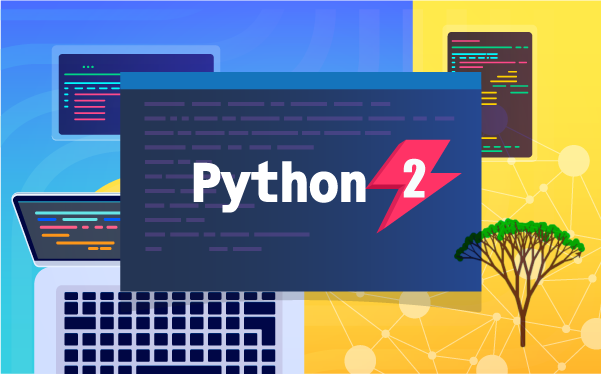« Middle School Courses
This course is included with our Coding/STEAM Curriculum - Middle School Plan# Python 201 Programming 300 Programming 201 Programming 202 Programming 301 Programming 302 JavaScript 101 Python 101 Web Development 101 Python 201 Drones 101 Augmented Reality micro:bit 101 MicroPython 101 Life Science 201 Physical Science 201 Earth Science 201 Math 201 Social Studies 201 English 201

A deeper look at Python for intermediate or advanced coders in upper middle or high school with a focus on real-world computing problems.

• Web

## Lesson Plan

### Introduction

In this lesson, students will explore recursion concepts and apply recursion as a problem-solving technique.

### Vocabulary

• Recursion: A problem-solving technique that breaks problems down into smaller problems until the problem cannot be made any smaller. Once the smallest version of the problem is solved, the next smallest version of the problem is solved with the answer from the smallest problem. This continues until the full problem has been solved.
• Base Case: The smallest instance of a problem. The problem cannot be broken down any further than a base case. Some recursion problems can have multiple base cases, like the Fibonacci sequence.
• Recursive Case: The problem expressed as a smaller instance of itself. For example, the recursive case for factorials is n * factorial(n-1). The problem of 5! can be broken down into 5 * 4! and then 5 * 4 * 3! and so on.
• Fractal: An infinite geometric pattern. Fractals can be drawn using recursion since they are a series of repeating patterns.
• Helper Function: A function outside of the main function that performs some of the actions. In the case of recursion, the main function usually calls a helper function which completes most of the computations.

### Objectives

Students will...
• Define recursion
• Write out what the base case and recursive case are for different mathematical problems
• Write programs that solve problems recursively, without loops

### Warm-Up(5 minutes)

• Describe a real-world problem that you can break down into smaller problems. Make sure to list and describe each section of the smaller problem.

### Activities(35 minutes)

Facilitate as students complete the Recursion modules on their own:
1. Recursion (Tutorial)
• Students will read a short document that explains recursion, which is a problem-solving technique where a solution is expressed as smaller instances of the problem.
• Check that students understand that “5!” can be expressed as “5 x 4 x 3 x 2 x 1.”
• Are students struggling with the “Integer sum” activity? Give a hint: Tell students to try using an “else” statement and “equal to” (==) operator as part of their solution.
• Tell students to click the “Next” button (located at the bottom of the document) to move on to the next module.
2. Multiple Base Cases (Tutorial)
• In this module, students will read a short document that explains how recursive solutions can have multiple base cases.
• Are students struggling with the “Exponent” puzzle? Give a hint: Tell students to use the code in the example as a reference. Also check that they are using the correct math operators.
3. Recursion and Lists (Tutorial)
• In this module, students will read a short document that explains recursion and lists.
• Are students struggling with the “Pascal’s Triangle” puzzle? Direct your students’ attention to the provided hint.
4. Fractal (Tutorial)
• In this module, students will read a short document that explains a fractal, which is an infinite geometric pattern. Additionally, students will use turtle graphics and recursion to draw a tree!
• Check that students are exploring the “Try This” section, which encourages students to change the argument of the function call of “tree().”
• Remind students to click the “Submit project” button--otherwise, you cannot see their “Recursion tree” solution!
5. Helper Function (Tutorial)
• In this module, students will read a short document that explains the helper function.
• This module does not contain puzzles.
• Optional: Ask students, “What does it mean to print all permutations of a string?” (print all possible re-orderings of the characters)
6. Quiz
• This quiz requires students to apply concepts from this lesson to solve 3 different puzzles. There are no multiple choice questions.

### Discussion Questions/Follow-Up Activities(20 minutes)

• Ask your students to write 2-3 sentences about something you feel proud of accomplishing in the Python 201 course.
• Ask your students to write 2-3 sentences about something they feel they can improve on related to coding and/or perseverance skills.

### US Standards

• CCSS-ELA: SL.8.1, RI.9-10.3, RI.9-10.6, RI.11-12.3, RI.11-12.6, L.9-10.3, L.9-10.6, L.11-12.3, L.11-12.6
• CCSS-Math: HSN.Q.A.1, HSN.Q.A.2, HSN.Q.A.3, MP.1, MP.2, MP.4
• CSTA: 2-AP-11, 2-AP-12, 2-AP-13, 2-AP-14, 2-AP-15, 2-AP-16, 2-AP-17, 3A-AP-17, 3B-AP-11, 3B-AP-12
• CS CA: 6-8.AP.11, 6-8.AP.12, 6-8.AP.13, 6-8.AP.14, 6-8.AP.15, 6-8.AP.16, 6-8.AP.17, 9-12.AP.12, 9-12.AP.14, 9-12.AP.16
• ISTE: 1.c, 1.d, 4.d, 5.c, 5.d

## Class Presentations

These student-facing slide presentations help educators seamlessly run Tynker lessons in a virtual or physical classroom setting. Each lesson has its own set of slides that introduce the big ideas, suggest unplugged activities, and include a section for each activity module. While running lesson slides, you can switch back and forth between the activity, the slides, answer keys and other lesson materials.Lesson 1
Welcome to Python
32 SlidesLesson 2
Variables and I/O
29 SlidesLesson 3
Data Types
29 SlidesLesson 4
Math Operators
32 SlidesLesson 5
Boolean Logic
35 SlidesLesson 6
Turtle Graphics
31 SlidesLesson 7
Branching
27 SlidesLesson 8
While Loops
27 SlidesLesson 9
Strings
32 SlidesLesson 10
Lists
28 SlidesLesson 11
For Loops
24 SlidesLesson 12
Functions
28 SlidesLesson 13
Dictionaries
20 SlidesLesson 14
Classes and Objects
24 SlidesLesson 15
Recursion
20 Slides